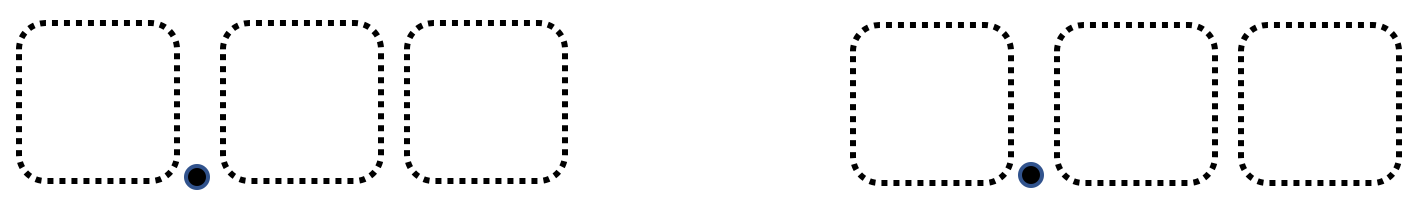# Greatest Difference of Two Decimal Numbers

Directions: Using the digits 0 to 9, at most one time each, fill in the boxes to create two numbers that both round to 5 and have the greatest (or least) possible difference with 5. Each digit may only be used once.### Hint

Where are the numbers that round to 5 on a number line?
How close does a number have to be to 5 to round to 5?

Greatest Difference: 4.60 and 5.39 with a difference of 0.79
Least Difference: 4.98 and 5.01 with a difference of 0.03

Source: Mike Wiernicki

## Equivalent Expressions with Fractions

Directions: Using the digits 0 to 9 at most one time each and choosing either …

1.I didn’t quite understand how to do this but ok

•2.4.60 and 5.12

3.4.4.60 and 5.39

5.It’s 4.32 and 5.01

6.What is this please tell me

7.8.9.Greatest Difference: 4.60 and 5.39 with a difference of 0.79
Least Difference: 4.98 and 5.01 with a difference of 0.03

10.ian molina torres

Greatest Difference: 4.60 and 5.39 with a difference of 0.79
Least Difference: 4.98 and 5.01 with a difference of 0.03

11.Greatest Different: 4.60 and 5.39 with a difference of 0.79 Least Difference: 4.98 with a difference of 0.03

12.Greatest Difference 4.60 and 5.39 with a difference of 0.79

Least Difference 4.98 with a difference of 0.03

13.4.98 and 3.76

14.Greatest Difference: 4.60 and 5.39 with a difference of 0.79
Least Difference: 4.98 and 5.01 with a difference of 0.03